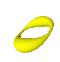Summer 2004

Mathematics Maths21a Summer 2004

Multivariable Calculus

Office: SciCtr 434
Email: knill@math.harvard.eduSend questions of potential general interest to maths21a@fas.harvard.edu.
 Question: When do classes start and what book will we used? Answer: Check out the syllabus page.
 Question: How many challenge problems do we have to do? Answer: To get full credit, you have to make serious progres on 15 of the problems.
 Question: What is the difference between Greens theorem and Stokes theorem? Answer: They are very similar. Both relate a line integral with a double integral. But Stokes deals with a three dimensional situation, while Green takes place in the plane. You can derive Greens theorem from Stokes theorem as we have seen in class.
 Question: How do I apply Stokes theorem for a surface without boundary? Answer: If there is no boundary, that line integral is zero. You can see this in two different ways. Take a closed surface like the sphere and cut out a little hole. The boundary is now a small circular curve around the hole. If you make the hole smaller, the corresponding line integral becomes smaller too. In the limit, when you have no curve any more, the line integral has vanished. An othe possibility is to cut the sphere into two halves and apply Stokes theorem for both halves. The two line integrals of the two boundaries are the same but have opposite sign. Again the line integral part is zero.
 Question: When will we know our grades. Answer: First, the exams have to be graded, which will take a while. The final exam solutions are online.